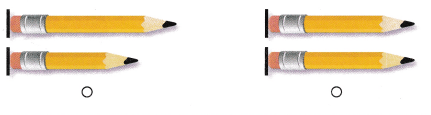# Texas Go Math Kindergarten Module 19 Assessment Answer Key

Refer to our Texas Go Math Kindergarten Answer Key Pdf to score good marks in the exams. Test yourself by practicing the problems from Texas Go Math Kindergarten Module 19 Assessment Answer Key.

## Texas Go Math Kindergarten Module 19 Assessment Answer Key

Concepts and Skills

Question 1.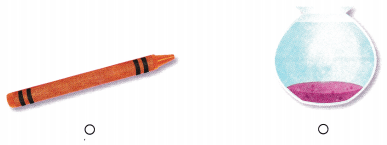Explanation:
The pot shows how much it holds
Pot is used to measure how much it holds
so, marked the pot.

Question 2.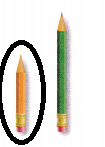Explanation:
The first pencil is smaller than the second pencil
so, circled the first pencil.

Question 3.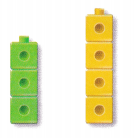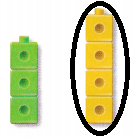Explanation:
The second tower is taller than the first tower
so, circled the second tower.

Question 4.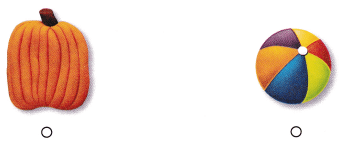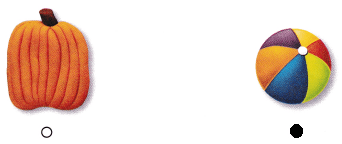Explanation:
The ball is lighter than the pumpkin
so, marked the ball.

Directions
1. Choose the correct answer. Which object would you most likely measure to find out how much it holds? TEKS K.7. 2. Circle the pencil that is shorter. TEKS K.7.B 3. Circle the cube tower flat is taller. TEKS K.7.B. 4. Choose the correct answer. Which object is lighter? TEKS K.7.B.

Question 5.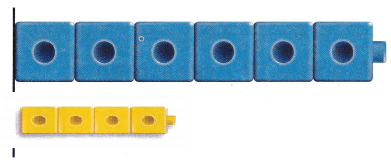Explanation:
The given cube train has 6 cubes
the cube train lesser than 6 cubes is 4 cubes
so, drawn 4 cubes train.

Question 6.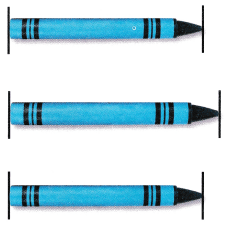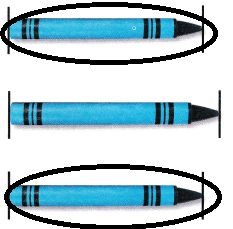Explanation:
The first crayon and the last crayons are of same length
so, circled the first and last crayons.

Question 7.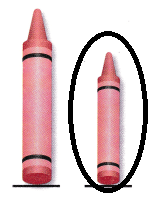Explanation:
The second crayon is shorter than the first crayon
so, circled the second crayon.

Question 8.
Texas Test Prep